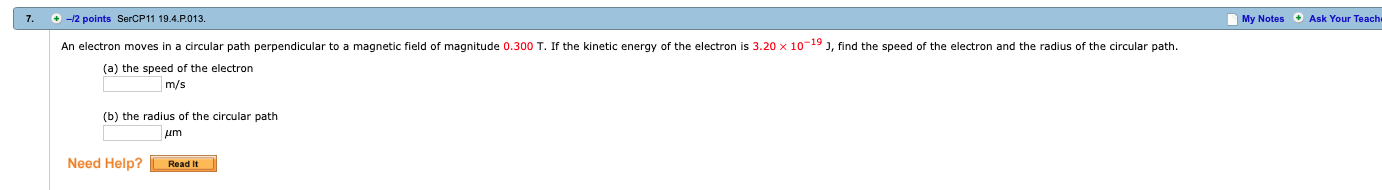7. +-2 points SerCP11 19.4.P.013.My Notes Ask Your TeachAn electron moves in a circular path perpendicular to a magnetic field of magnitude 0.300 T. If the kinetic energy of the electron is 3.20 × 10-19 J, find the speed of the electron and the radius of the circular path.(a) the speed of the electronm/s(b) the radius of the circular pathNeed Help?Readit

Questionhelp_outlineImage Transcriptionclose7. +-2 points SerCP11 19.4.P.013. My Notes Ask Your Teach An electron moves in a circular path perpendicular to a magnetic field of magnitude 0.300 T. If the kinetic energy of the electron is 3.20 × 10-19 J, find the speed of the electron and the radius of the circular path. (a) the speed of the electron m/s (b) the radius of the circular path Need Help?Readit fullscreen
Step 1

(a)

The speed of electron is,

Step 2

(b)

The radius of circu...

Want to see the full answer?

See Solution

Want to see this answer and more?

Our solutions are written by experts, many with advanced degrees, and available 24/7

See Solution
Tagged in

Electric Charges and Fields